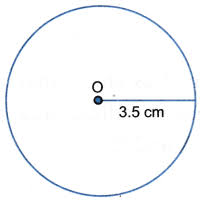# Java Program Calculate Perimeter Of Circle | 4 Simple Ways

Java program to calculate the perimeter of a circle – In this specific blog post, we are going to discuss the numerous means to find out the perimeter of a circle in Java Programming.

Proper examples and sample programs have been added so that people can grasp the logic and meaning behind the said codes in java programming.

The many methods used to decipher the perimeter of a circle in this piece are as follows:

• Using Scanner Class
• Using Command Line Arguments
• Using Static Function

As it is clearly known, a circle is the collection of infinite points which form a locus equidistant from a single point. The locus is known as the circumference of the circle.

The single point within the circle is known as the centre of the circle. The distance between the centre of the circle and the circumference is regarded as the radius of the circle.As you can see, this is a circle with a radius of 3.5cm. Also, the line which passes through the centre of a circle and connects the circumference on both sides of the circle is said to be the diameter of the circle.

The ratio between to the Circumference of a circle and the Diameter is always equal to Pi (22/7).

Hence, the Circumference of the above circle is as follows:

Circumference = Pi * D = 2 * r * Pi

= 2 * 3.5 * Pi

= 22cm.

Thus, the multitude of means to calculate the circumference of a circle in Java programming is as follows:

Output:

Output:

Output:
x

## Plus Star Pattern Java Program | Patterns

Java program to print plus star pattern program – We have written the below print/draw ...